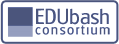# Stuybash.org

## Search Results

• ### #349Up↑+103/125Down↓[Report]2009-01-26 17:30 EST

Dr. Chen: Then at end we get simple integral!
*writes on board*
Integral from 0 to 2 of 3cos^2(theta)sin(theta)(-sin(theta))d(theta) + 12sin(theta)cos^2(theta)(3cos(theta))d(theta) + 2cos(theta)e^(cos^2(theta))(-2sin(theta))d(theta)
Dr. Chen: That's it!